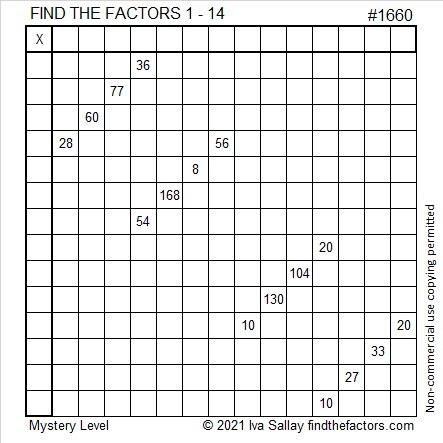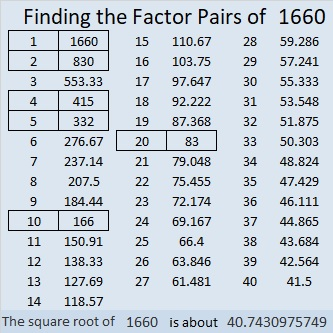# 1660 A 14×14 Mystery Puzzle

Contents

### Today’s Puzzle:

Adding a few more factors to the multiplication table really complicates this mystery-level puzzle. For example, will the common factor of 28 and 56 be 4, 7, or 14? If it were just a 10 × 10 or a 12 × 12 puzzle, answering that question would be easy. Not so with a 14 × 14 puzzle. Remember to use logic on every step while you find its unique solution.You can print the puzzle or type the solution on this excel sheet: 10 Factors 1650-1660 with Taxman Scoring Calculator

### Factors of 1660:

• 1660 is a composite number.
• Prime factorization: 1660 = 2 × 2 × 5 × 83, which can be written 1660 = 2² × 5 × 83.
• 1660 has at least one exponent greater than 1 in its prime factorization so √1660 can be simplified. Taking the factor pair from the factor pair table below with the largest square number factor, we get √1660 = (√4)(√415) = 2√415.
• The exponents in the prime factorization are 2, 1, and 1. Adding one to each exponent and multiplying we get (2 + 1)(1 + 1)(1 + 1) = 3 × 2 × 2 = 12. Therefore 1660 has exactly 12 factors.
• The factors of 1660 are outlined with their factor pair partners in the graphic below.### More About the Number 1660:

1660 is the hypotenuse of a Pythagorean triple:
996-1328-1660, which is (3-4-5) times 332.

This site uses Akismet to reduce spam. Learn how your comment data is processed.